# Revision Notes on Magneto-static and Magnetic Effect of Current

• Magneto-static:- It is the study of magnetic fields in systems where the currents are steady (not changing with time). It is the magnetic analogue of electrostatics, where the charges are stationary.

• Magnitude of magnetic force between two poles (Coulomb’s law in magnetism):-

F = (µ0/4π) (m1m2/r2)

Here µ0 is called the absolute magnetic permeability of free space.

µ0= 4π×10-7 Wb A-1 m-1

• Magnetic field:- Magnetic field, of any magnetic pole, is the region (space) around it in which its magnetic influence can be realized.

• Lines of Force (Flux Lines):- Line of force is the path along which a unit north pole would move if it were free to do so.

• Properties of magnetic lines of force:-

(a) Lines of force are directed away from a north pole and are directed towards a south pole. A line of force starts from a north pole and ends at a south  pole if they are isolated poles.

(b) Tangent, at any point, to the magnetic line of force gives the direction of magnetic intensity at that point.

(c) Two lines of force never cross each other.

• Magnetic Dipole:- A combination of two isolated , equal and opposite magnetic poles separated by a small distance constitutes a magnetic dipole.

• Magnetic Moment:- Magnetic moment M of a magnetic dipole is defined as the product of its pole strength and the magnetic length. M = m×2l

• Torque in Magnetic field:- ?

• Work done in rotating a magnetic dipole in a magnetic field:- W = MB (cosθ1 – cosθ2)

• Potential Energy of a magnetic dipole in a magnetic field:-

• Magnetic moment (M):- M = I×A

• Other formulae of M:-

(a) M = nIπr2

(b) M= eVr/2 = er2ω/2 = er2f/2 = er2 π/T

(c)  M = B

• Resultant magnetic moment :-

(a) When two bar magnets are lying mutually perpendicular to each other, then, M = √[M12+M22= √2 mpI(b) When two coils, each of radius r and carrying current i, are lying concentrically with their planes at right angles to each other, then M = √[M12+M22= [√2Ir2    If M1 = M2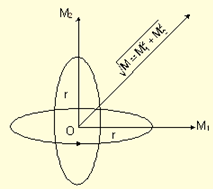• Atoms as a magnetic dipole:-

(a) I = /2π

(b) M = eωr2/2

(c) M = n (eh/4πm)

The term eh/4πm is called Bohr’s magneton. It is the smallest value of magnetic moment which an electron can possesss.

• Magnetic flux density at a distance from a magnetic dipole in free space:-  B= (µ0/4π) (m1/r2)

• Force:- F = (µ0/4π) (m1 m2/r2)

• Magnetic intensity at any point due to a magnetic pole in free space:- F = (µ0/4π) (m/r2)

• Magnetic intensity due to a bar magnet in free space:-

(a) Point situated on the axial line (End-on position):-

F = (µ0/4π) [2Mr/(r2-l2)2]

In case of a magnetic-dipole, F = (µ0/4π) [2M/r3]

(b) Point situated on equatorial line (Broad side-on position):-

F = (µ0/4π) [M/(r2+l2)3/2]

In case of a magnetic-dipole, F = (µ0/4π) [M/r3]

(c) Point situated anywhere:-

F= (µ0/4π) [M/r3] √1+3 cos2θ

Direction, tan β = ½ tan θ

• Combined magnetic field due to bar magnet and earth – “Neutral Points”:-

Bar magnet placed in a magnetic meridian:-

(a) North pole facing north of earth:-

B = (µ0/4π) [M/(r2+l2)3/2]

At neutral points, B=H

So, H = (µ0/4π) [M/(r2+l2)3/2]

(b) North pole facing south of earth:-

B = (µ0/4π) [2Mr/(r2-l2)2]

At neutral points, B=H

So, H = (µ0/4π) [2Mr/(r2-l2)2]

• Intensity of magnetization (I):-

Intensity of magnetization (I), is defined as the magnetic moment (M) developed per unit volume (V) of the specimen, when subjected to a uniform magnetic field.

I = M/V = m/a

Here m is the pole strength and a is the area of the specimen.

• Relation between magnetic field (B) and field intensity (H):- B = H+4πI

• Permeability (µ):- It is defined as the ratio between magnetic induction to the strength of magnetic field. µ = B/H

(a) For paramagnetic and ferromagnetic substances, B > H. So, µ > 1

(b) For diamagnetic substances, B < H. So, µ < 1

• Susceptibility (k):- Susceptibility of a magnetic substance is defined as the ratio between intensity of magnetization (I) to the strength of magnetic field (H). k = I/H

• Relation between µ and k:- µ = 1+4πk

• For substances which get magnetized in the direction of magnetic field,

(a) I is positive

(b) k is positive

(c) B > H

(d) µ > 1

• For substances which get magnetized in the direction opposite to that of magnetic field,

(a) I is negative

(b) k is negative

(c) B < H

(d) µ < 1

• Magnetic Substance:- A substance which is affected by a magnetic field is called a magnetic substance.

(a) Diamagnetic substances:- Diamagnetic substances are those substances which are repelled by the magnets.

Example- antimony, bismuth, lead, tin, zinc, mercury, gold, phosphorus

(b) Paramagnetic substances:- Paramagnetic substances are those substances which are weakly attracted by the magnets.

Example-aluminium, platinum, oxygen, manganese, chromium

(c) Ferromagnetic substances:- Ferromagnetic substances are those substances which are strongly attracted by the magnets.

Example- iron, cobalt, nickel

• Curie-Weiss Law:-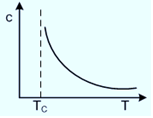χ – T Curve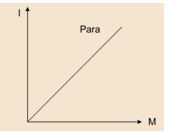I-M curve for paramagnetic material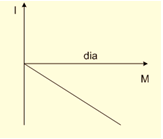I-M curve for diamagnetic material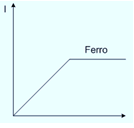I-M curve for ferromagnetic materials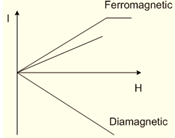I-H curve
• Magnetic flux:-

Magnetic flux lined with the surface is defined as the product of area and component of B perpendicular that area.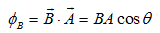and

?B = µnAH

Here, µ is the permeability of the medium, n is the number of turns, A is the area and H is the magnetic field intensity.

(a) When θ = 90º, cosθ = 0. So, ?B = 0

This signifies, no magnetic flux is linked with surface when the field is parallel to the surface.

(b) When θ = 0º, cosθ = 1. So,( ?B)max = 1

This signifies, magnetic flux linked with a surface is maximum when area is held perpendicular to the direction of field.

• Biot-Savart Law or Ampere’s Theorem:-Or, dB = (µ0/4π) (I dl sin θ/r2)

• Field due to straight current carrying conductor of finite length at a point P, perpendicular distance a from the linear conductor XY:-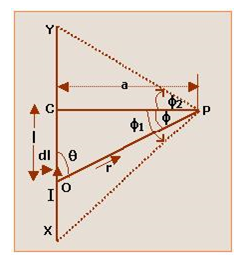B =  (µ0I/4πa)× (sin ?1 + sin ?2)

Direction:-

(a) For current in the conductor from X to Y, the direction of B is normal to the plane of    conductor downwards.

(b) For current in the conductor from Y to X, the direction of B is normal to the plane of    conductor downwards.

• Field due to straight carrying conductor of infinite length at a point P, perpendicular distance R from the linear conductor XY:-

B = (µ0I/2πa) (Direction is same as given above)

• Field due to two concentric coils of radii r1 and r2 having turns N1 and N2 in which same current I is flowing in anticlockwise direction at their common center O:-B = µ0 I/2 [N1/r1+ N2/r2]

If the number of turns in them is same, B = µ0 NI/2 [1/r1+ 1/r2]

Direction:- Direction of B will be normal to the plane of paper upwards.

• Field due to two concentric coils of radii r1 and r2 having turns  N1 and N2 in which same current I is flowing in mutually opposite direction at their common center O:-B = µ0 I/2 [N1/r1- N2/r2]

If the number of turns in them is same, B = µ0NI/2 [1/r1- 1/r2]

Direction:- Direction of B will be normal to the plane of paper upwards.

• Field due to circular coil at the center O :-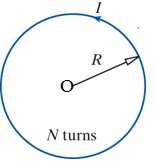B = µI/2R

• Field due to two parallel very long linear conductors carrying current in same direction:-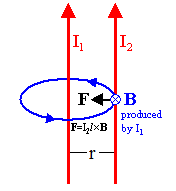(a) At point P i.e. at a distance r/2 from both conductors, B=0

(b) At a point Q i.e. at a distance x from first and r+x from second conductor, B =µ0 2I/4π [(1/x) + (1/r+x)]

Direction:- B is normal to the plane of paper downwards.

(c) At a point P i.e. at a distance x from first and r-x from second conductor,

B =µ0 2I/4π [(1/x) - (1/r-x)]

If B is positive, then its direction will be normal to the plane of paper upwards.

If B is negative, then its direction will be normal to the plane of paper downwards.

• Field due to two parallel very long linear conductors carrying current in opposite direction (refer above figure):-

(a) At point P distance x from first conductor, B = µ0 2I/4π [(1/x) + (1/r-x)]

Direction:- of B will be normal to the plane of paper downwards.

(b) At point Q distance x from first conductor, B = µ0 2I/4π [(1/x) - (1/r-x)]

Direction:- of B will be normal to the plane of paper upwards.

• Field due to semicircular arc of wire at the center O of the arc:- B = (µ0 /4π) (πI/r)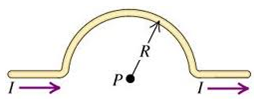Direction:- Direction of B will be at right angle to the plane of circular arc downwards. If the direction current is in anticlockwise, then the direction of field B will be a right angle to the plane of circular arc upwards.

• Field due to straight wire and loop at the center O of the loop (If the current in the looop in anticlockwise direction):- B = (µ0 /4π) [2πI/r + 2I/r]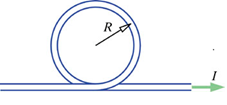Direction:- Normal to the plane of paper upwards.

• Field due to straight wire and loop at the center O of the loop(If the current in the looop in clockwise direction):- B = (µ0 /4π) [2πI/r - 2I/r]Direction:- Normal to the plane of paper downwards

• Field due to two semicircular arc of wire:-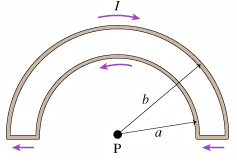?B = µ0I/4 [1/a-1/b]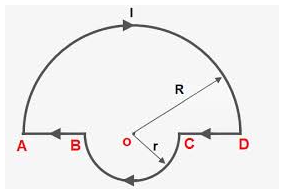B = µ0I/4 [1/R+1/r]

Direction:- Normal to the plane of paper downward.

• Field due to two concentric circular arcs at O:-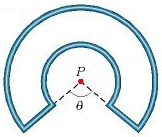B = (µ0/4π) [1/r1-1/r2]

Here r1 is the radius of inner circle and r2 is the radius of outer circle.

Direction:- Normal to the plane of paper upwards

• Field due to semicircular arc and straight conductor at point P:-B = (µ0I/4πr) [π+2]

Direction:- Normal to the plane upward.

• Field due to semicircular arc and straight conductor at point O:- B = (µ0I/4πr) [π+1]

Direction: Normal to the plane upward.

• Field due to square loop having length of side a at center C:-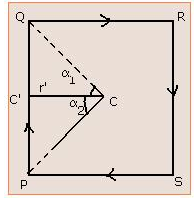B = 2√2(µ0I/ πa)

Direction:- Normal to the plane of paper downwards.

• Field at the center of a circular coil carrying current I:-B = (µ0/2) (NI/r)

• Magnetic field at any point on the axis of a circular coil carrying current I:-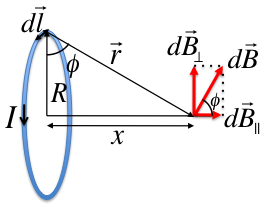B = (µ0/2) [NIa2/(a2 +x2)3/2]

(a) Magnetic field at the center of the coil:- B = (µ0/2) [NI/a]

(b) Magnetic field at a point situated large distance away on the axis:- B = (µ0/2π) [NIA/x3]

(c) Current loop as a magnetic dipole:- B = (µ0/4π) [2M/x3]

Here, M (=IA) is the magnetic moment of the magnetic dipole.

• Magnetic field at any point on the axis of a solenoid carrying current:-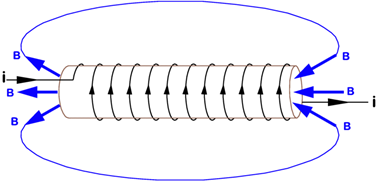B = (µ0NI/2l) [cos?1-cos ?2]

For an infinitely long solenoid, ?1 = 0 and ?2 = π. So, B=µ0NI

At one end, B = µ0NI/2

• Field due to a current in cylindrical rod:-

?(a) Outside:- B = µ0I/2πR

(b) Surface:- B = µ0I/2πR

(c) Inside:- B = µ0IR/2πR2

• Field due to a toroid:-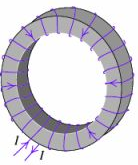(a) Inside:- B = µ0NI - µ0NI/2πR

(b) Outside:- B =0

• Force on electric current:-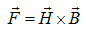• Force on a moving charge in a magnetic field:-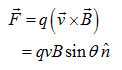• Lorentz Force:-• Motion of a charged particle at right angles to a magnetic field:- Radius, r = mv/qB

• Force on a conductor carrying current and placed in a magnetic field:-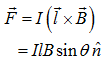• Fleming’s left hand rule:- Stretch first finger, central finger and the thumb of your left hand in mutually perpendicular directions. If the first finger points towards magnetic field, central finger points towards electric current then the thumb gives the direction of force acting on the conductor.• Force between two parallel conductors carrying currents:-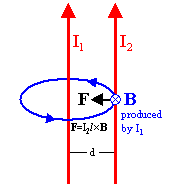F = µ0I1I2l /2πd

• Torque on a current loop:- = NIBA cos θ = NMB cos θ     (Since, M = IA)

• Moving Coil Galvanometer:- I = (C/nBA)θ  =

Here K = C/nBA is known as the reduction factor of the moving coil galvanometer.

• Sensitivity of a Galvanometer:-

(a) Current Sensitivity:- Si = C/nAH

Smaller the value of Si, more sensitive is the galvanometer.

(b) Voltage Sensitivity:- Sv =V/G = CG/nAH

Smaller the value of Sv, more sensitive is the galvanometer

• Conversion of a galvanometer into an ammeter:-

(a) Is/Ig = G/S

(b) S=GIg/Ig = GIg/I-Ig

• Conversion of a galvanometer into a voltmeter:- R =(V/Ig) – G

• Ampere’s current law:-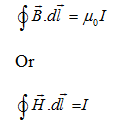• Cyclotron:-

(a) T = 2πm/qB

(b) v = qB/2πm

(c) ω = θB/m

(d) radius of particle acquiring energy E, r = (√2mE)/qB

(e) velocity of particle at radius r, v = qBr/m

(f) the maximum kinetic energy (with upper limit of radius = R)

Kmax = ½ [q2B2R2/m]

• Magnetic field produced by a moving charge:-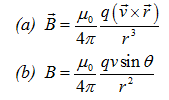### Course Features

• Video Lectures
• Revision Notes
• Previous Year Papers
• Mind Map
• Study Planner
• NCERT Solutions
• Discussion Forum
• Test paper with Video Solution

r